Systems of EquationsOverview

Expect to see some questions on the topic of Linear Systems on the SAT. In this section, we will review the following aspects of this topic:

• Definition of a Linear System
• Solving a Linear System
• Substitution Method
• Elimination Method
• Graphical Method

Let’s look at each of these in more detail:

Definition of a Linear System

A linear system involves two or more linear equations that represent graphs of linear functions. Where the graphs intersect is the solution to the system.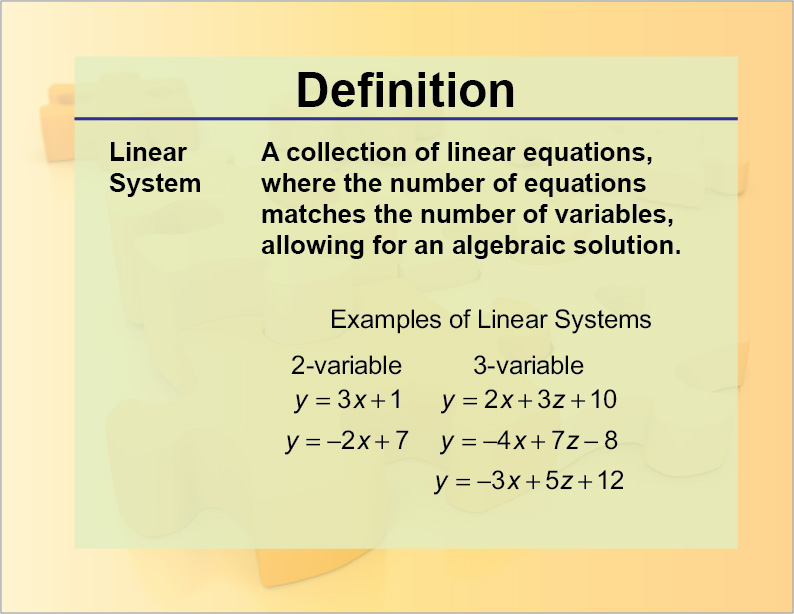The kind of linear systems questions you will find on the SAT involve two variables. Here is an example of a linear system with the solution highlighted.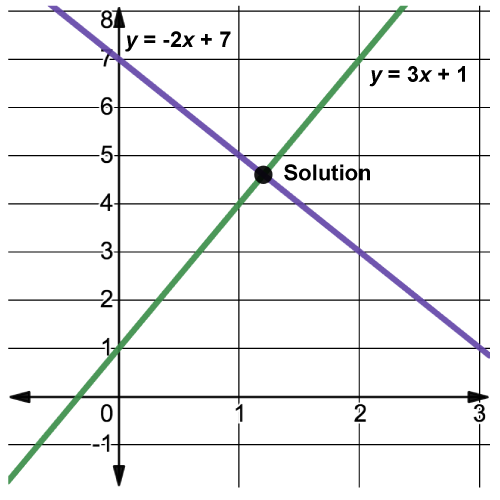If a linear system doesn’t have a solution, that means the graphs of the lines are parallel.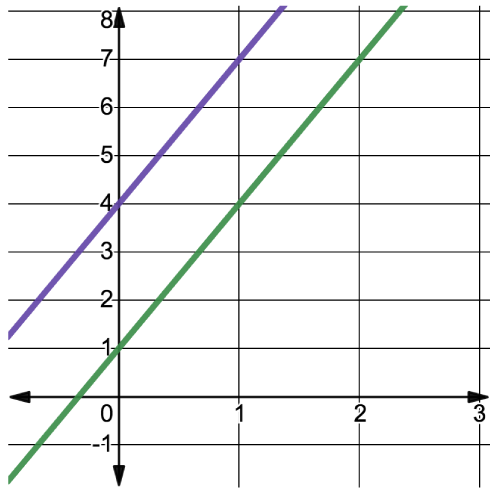Lines that are parallel have the same slope.

Solving a System Using the Substitution Method

In this section we go over the process of solving a linear system using the Substitution Method. This method is summarized below.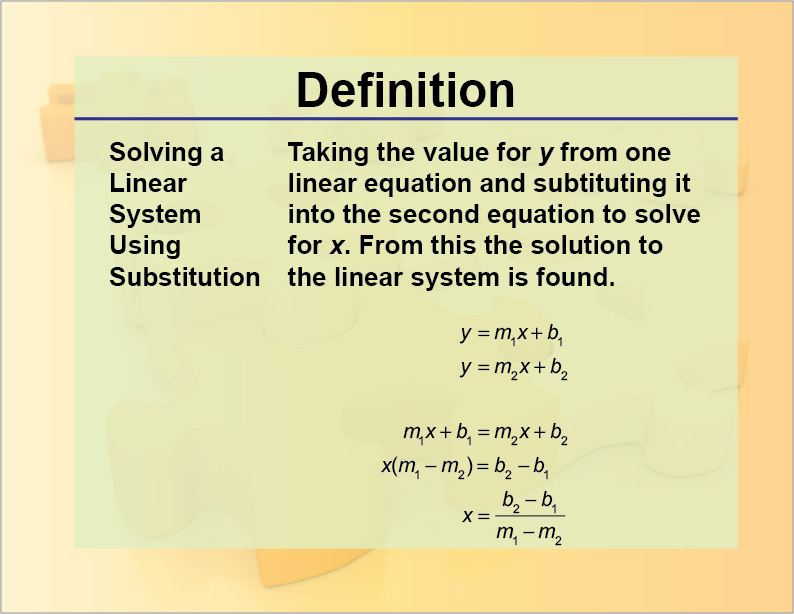SAT Skill: Solving Systems Using Substitution Example 1 What is the solution to this system of equations?Substitute y in one of the equations with the corresponding expression with x. Then solve for x.Plug in the value for x to find y:The solution to the system is (-5, -25). Example 2 What is the solution to this system of equations?Rewrite one equation to get a substitution value for y and plug that into the second equation. Then solve for x:Plug in the value for x to find y:The solution to the system is (1, -6).

To see examples of solving systems using the Substitution Method, click on this link.

Solving a Linear System Using the Elimination Method

In this section we go over the process of solving a linear system using the Elimination Method. This method is summarized below.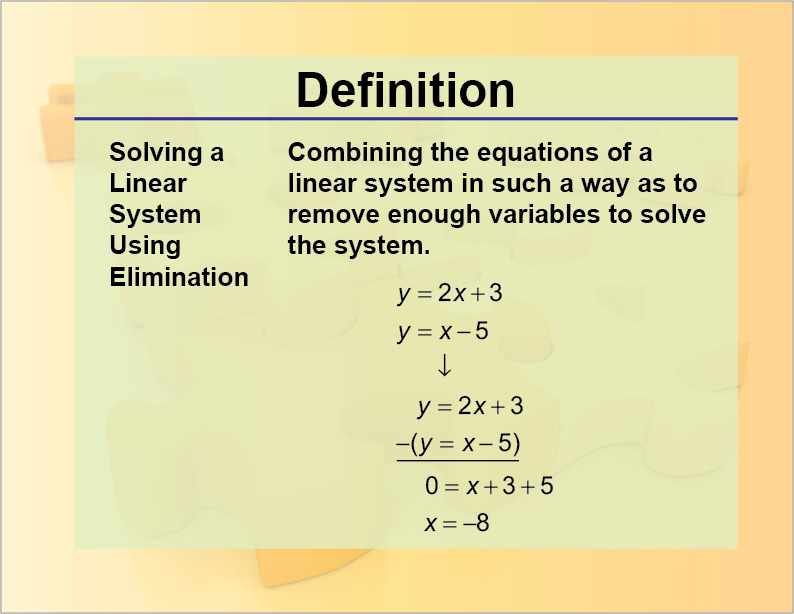SAT Skill: Solving Systems Using Elimination Example 1 What is the solution to this system of equations?Multiply one of the equations by a factor such that when both equations are added, one of the variables is eliminated. Solve for the remaining variable.Plug in the value for x to find y:The solution to the system is (-2, 4).

To see examples of solving systems using the Elimination Method, click on this link.

Solving a System Using a Graphical Approach

In this section we go over the process of solving a linear system using the Graphical Method. This method is summarized below.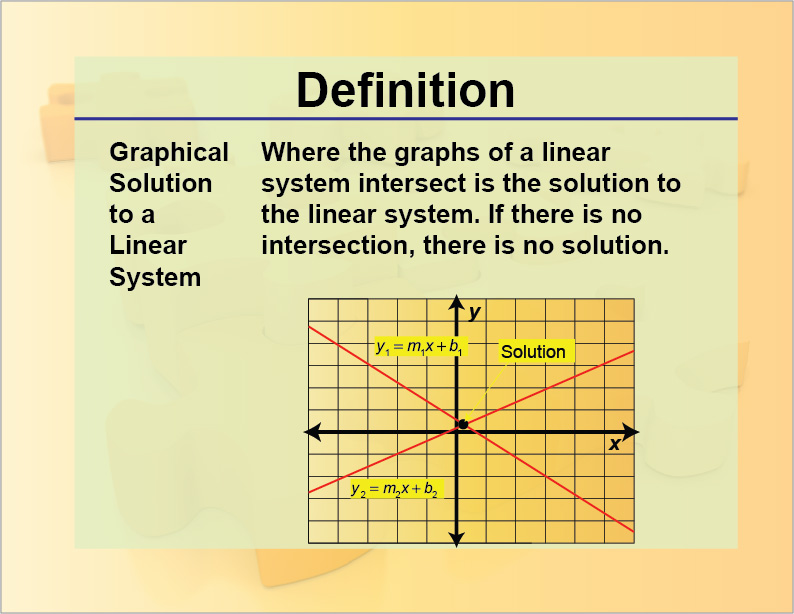SAT Skill: Solving Systems Using the Graphical Method Example 1 What is the solution to this system of equations?Graph the linear functions and find the point of intersection: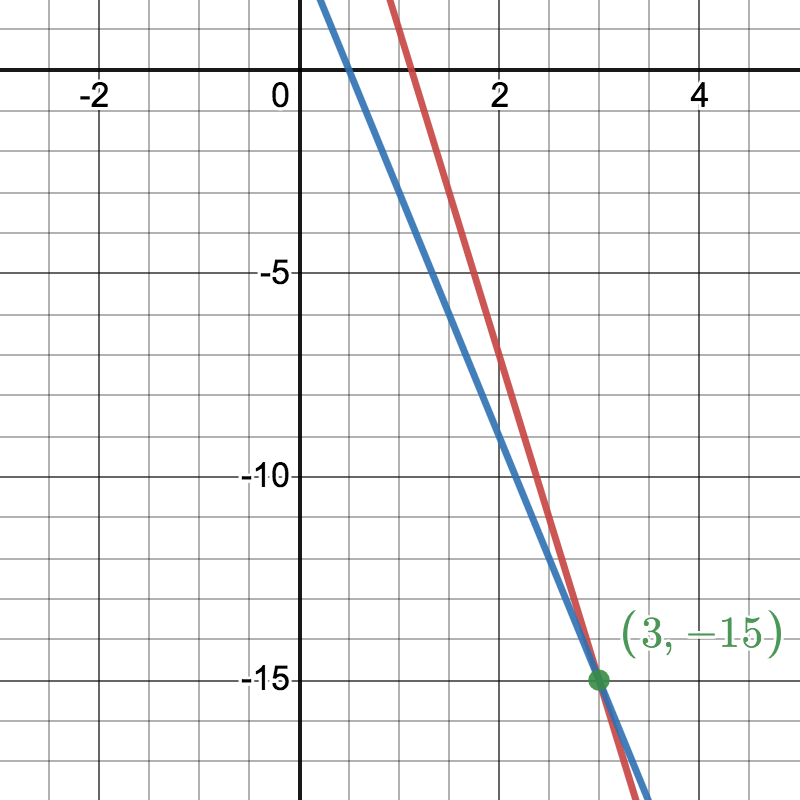The solution to the system is (3, -15).

To see examples of solving linear systems by graphing, click on this link